# Celebrating 1500 with a Horse Race and Much More!

Writing 1500 posts is quite a milestone. I’ll begin the celebration with an exciting horse race! Let me explain:

Each prime number has exactly 2 factors. Every composite number between 1401 and 1500 has somewhere between 4 and 36 factors. Which quantity of factors do you think will appear most often for these numbers? Pick that amount as your pony and see how far it gets in this horse race!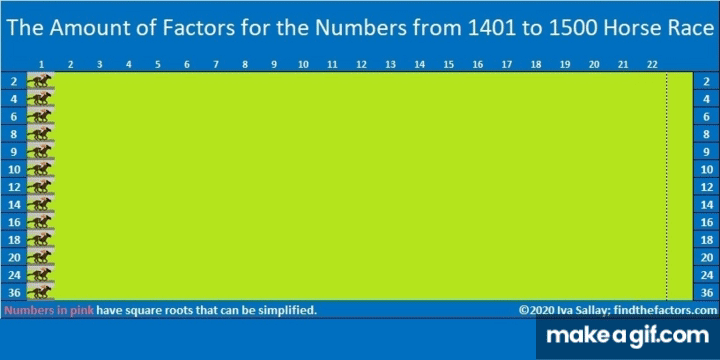make science GIFs like this at MakeaGif

Did the race results surprise you? They surprised me!

### Prime Factorization for numbers from 1401 to 1500:

Here’s a chart showing the prime factorization of all those numbers and the amount of factors each number has. Numbers in pink have exponents in their prime factorization so their square roots can be simplified:### Today’s Puzzles:

Let’s continue the celebration with a puzzle: 1500 has 12 different factor pairs. One of those pairs adds up to 85 and one of them subtracts to give 85. Can you find those factor pairs that make sum-difference and write them in the puzzle? You can look at all of the factor pairs of 1500 in the graphic after the puzzle, but the second puzzle is really just the first puzzle in disguise. So try solving that easier puzzle first.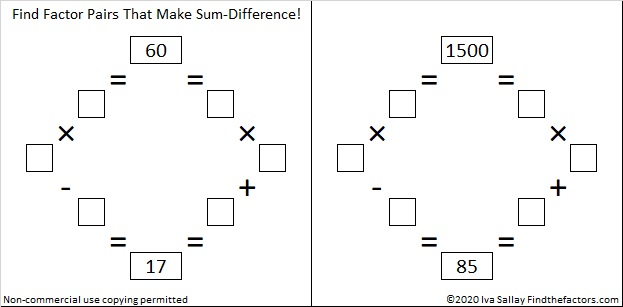### Factors of 1500:

• 1500 is a composite number.
• Prime factorization: 1500 = 2 × 2 × 3 × 5 × 5 × 5, which can be written 1500 = 2² × 3 × 5³
• 1500 has at least one exponent greater than 1 in its prime factorization so √1500 can be simplified. Taking the factor pair from the factor pair table below with the largest square number factor, we get √1500 = (√100)(√15) = 10√15
• The exponents in the prime factorization are 2, 1, and 3. Adding one to each exponent and multiplying we get (2 + 1)(1 + 1)(3 + 1) = 3 × 2 × 4 = 24. Therefore 1500 has exactly 24 factors.
• The factors of 1500 are outlined with their factor pair partners in the graphic below.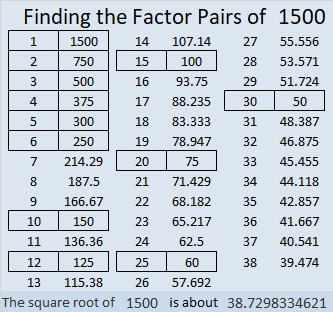### A Forest of Factor Trees:

Take a few minutes to hike in this forest featuring a few of the MANY possible factor trees for 1500. Celebrate each tree’s uniqueness!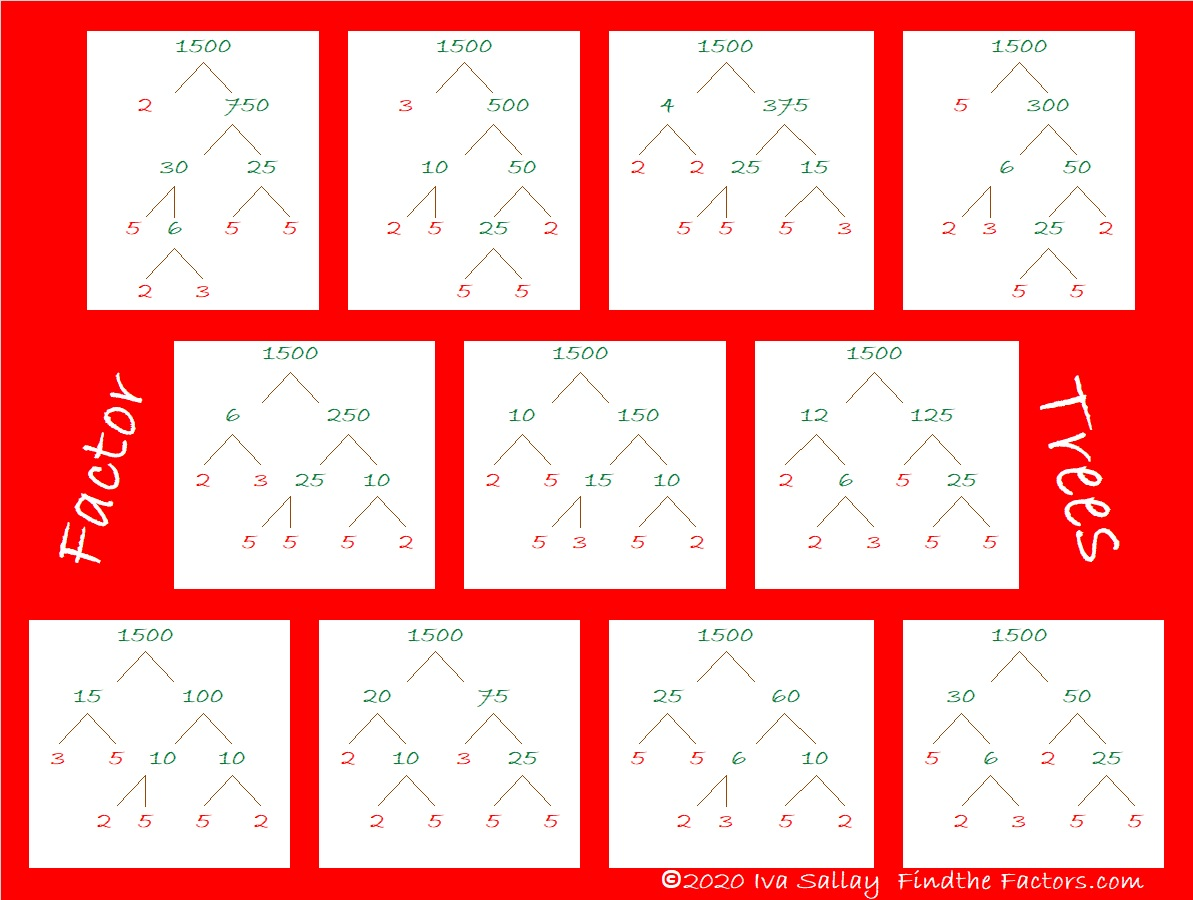### Other Facts to Celebrate about 1500:

Oeis.org tells us that  (5+1) × (5+5) × (5+0) × (5+0) = 1500.

1500 is the hypotenuse of THREE Pythagorean triples:
420-1440-1500, which is (7-24-25) times 60,
528-1404-1500, which is 12 times (44-117-225),
900-1200-1500, which is (3-4-5) times 300.

# 1332 Yet Another Christmas Tree

Here is yet another Christmas tree for you to enjoy this holiday season.

Print the puzzles or type the solution in this excel file:10-factors-1321-1332

Here are a few facts about the number 1332:

• 1332 is a composite number.
• Prime factorization: 1332 = 2 × 2 × 3 × 3 × 37, which can be written 1332 = 2² × 3² × 37
• The exponents in the prime factorization are 2, 2 and 1. Adding one to each and multiplying we get (2 + 1)(2 + 1)(1 + 1) = 3 × 3 × 2 = 18. Therefore 1332 has exactly 18 factors.
• Factors of 1332: 1, 2, 3, 4, 6, 9, 12, 18, 36, 37, 74, 111, 148, 222, 333, 444, 666, 1332
• Factor pairs: 1332 = 1 × 1332, 2 × 666, 3 × 444, 4 × 333, 6 × 222, 9 × 148, 12 × 111, 18 × 74 or 36 × 37
• Taking the factor pair with the largest square number factor, we get √1332 = (√36)(√37) = 6√37 ≈ 36.49658

Here are a couple of factor trees for 1332:Since 36 × 37 = 1332, we know that 1332 is the sum of the first 36 even numbers. (The first 36 numbers add up to the infamous 666, and 2 times 666 is 1332.)

Because 1332 is divisible by both 3 and 37, it has several repdigits as factors, 111, 222, 333, 444, and 666.

1332 is the sum of four consecutive prime numbers:
317 + 331 + 337 + 347 = 1332

1332 is the sum of two square numbers:
36² + 6² = 1332

1332 is the hypotenuse of a Pythagorean triple:
432-1260-1332 which is 36 times (12-35-37)
It can also be calculated from 2(36)(6), 36² – 6², 36² + 6²

# 1320 Christmas Factor Trees

### Today’s Puzzle:

Regardless of its size, an evergreen tree is a mighty symbol at Christmastime. Today’s factoring puzzle features a couple of relatively small Christmas trees, but don’t think for even one minute that these little trees make for an easy puzzle. It’s a level 6 puzzle so there are several places that the clues might trick you. Use logic throughout the entire process, and you should be able to solve it!

Print the puzzles or type the solution in this excel file: 12 factors 1311-1319

### Factor Trees for 1320:

That factoring puzzle has nothing to do with the factors of 1320. In case you are looking for factor trees for the number 1320, here are a few of the MANY possible ones:

### Factors of 1320:

• 1320 is a composite number.
• Prime factorization: 1320 = 2 × 2 × 2 × 3 × 5 × 11, which can be written 1320 = 2³ × 3 × 5 × 11.
• The exponents in the prime factorization are 3, 1, 1, and 1. Adding one to each and multiplying we get (3 + 1)(1 + 1)(1 + 1) )(1 + 1) = 4 × 2 × 2 × 2 = 32. Therefore 1320 has exactly 32 factors.
• Factors of 1320: 1, 2, 3, 4, 5, 6, 8, 10, 11, 12, 15, 20, 22, 24, 30, 33, 40, 44, 55, 60, 66, 88, 110, 120, 132, 165, 220, 264, 330, 440, 660, 1320
• Factor pairs: 1320 = 1 × 1320, 2 × 660, 3 × 440, 4 × 330, 5 × 264, 6 × 220, 8 × 165, 10 × 132, 11 × 120, 12 × 110, 15 × 88, 20 × 66, 22 × 60, 24 × 55, 30 × 44 or 33 × 40
• Taking the factor pair with the largest square number factor, we get √1320 = (√4)(√330) = 2√330 ≈ 36.331804

### Sum-Difference Puzzles:

330 has eight factor pairs. One of those pairs adds up to 61, and  another one subtracts to 61. Put the factors in the appropriate boxes in the first puzzle.

1320 has sixteen factor pairs. One of the factor pairs adds up to ­122, and a different one subtracts to 122. If you can identify those factor pairs, then you can solve the second puzzle!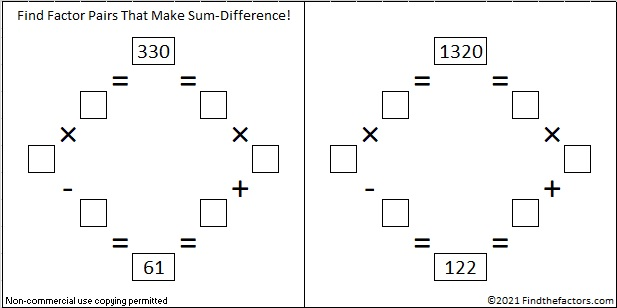The second puzzle is really just the first puzzle in disguise. Why would I say that?

1320 is the third number that is at the top of more than one Sum-Difference Puzzle. This next one is a primitive.

Again, 1320 has sixteen factor pairs. One of the factor pairs adds up to 73, and a different one subtracts to 73. If you can identify those factor pairs, then you can solve this puzzle!If you would like a little help finding those factor pairs that make sum-difference, the chart below lists all of 1320’s factor pairs with their sums and their differences.### More about the Number 1320:

1320 is the sum of consecutive primes FOUR different ways:
It is the sum of the eighteen primes from 37 to 109,
107 + 109 + 113 + 127 + 131 + 137 + 139 + 149 + 151 + 157 = 1320,
149 + 151 + 157 + 163 + 167 + 173 + 179 + 181 = 1320, and
659 + 661 = 1320.

1320 is the hypotenuse of a Pythagorean triple:
792-1056-1320 which is (3-4-5) times 264.

# 1296 Last Digit Effort

If you always use a calculator to find the roots of perfect powers like 1296, then you will likely miss out on discovering the wonderful patterns those powers have. Look at the table below. What do you notice? What do you wonder?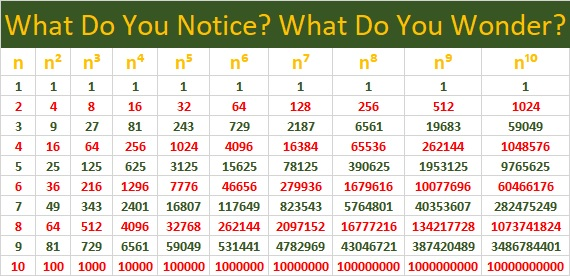When I looked at it, I noticed several patterns and I wondered about a few things that I explored to see if they were true. However, I won’t tell you what I noticed or what conjectures I made until the end of this post, because I don’t want to spoil YOUR chance to discover those patterns yourself. I will tell you that there are MANY patterns in the table above and that most of my conjectures were true, but one of them turned out not to be.

Besides the patterns that you will have to discover for yourself, the table can be very useful: You can use the table to find several fractional powers of the numbers in the body of the table. For example, if you wanted to find out what is 1296^(7/4), then you could simply run your finger down the n⁷ column and the n⁴ column simultaneously until you reach 1296 on the n⁴ column (because 4 is the denominator of the fractional power). Your answer, 279936, will be in the n⁷ column on the same row as 1296.

However, if you wanted to find 1296^(3/2), you would either have to expand the table to include 36² and 36³, or you would have to use the fact that 3/2 = 6/4. Then you could use the process of the previous paragraph to see that
1296^(3/2) = 1296^(6/4) = 46656.

Now I’ll share some more facts about the number 1296:

• 1296 is a composite number, a perfect square, and a perfect fourth power.
• Prime factorization: 1296 = 2 × 2 × 2 × 2 × 3 × 3 × 3 × 3, which can be written 1296 = 2⁴ × 3⁴
• The exponents in the prime factorization are 4 and 4. Adding one to each and multiplying we get (4 + 1)(4 + 1) = 5 × 5 = 25. Therefore 1296 has exactly 25 factors.
• Factors of 1296: 1, 2, 3, 4, 6, 8, 9, 12, 16, 18, 24, 27, 36, 48, 54, 72, 81, 108, 144, 162, 216, 324, 432, 648, 1296
• Factor pairs: 1296 = 1 × 1296, 2 × 648, 3 × 432, 4 × 324, 6 × 216, 8 × 162, 9 × 144, 12 × 108, 16 × 81, 18 × 72, 24 × 54, 27 × 48, or 36 × 36
• Taking the factor pair with the largest square number factor, we get √1296 = (√36)(√36) = 36.1296 is the smallest number with exactly 25 factors.

There are MANY different factor trees you could make for 1296, but here are some made with squares: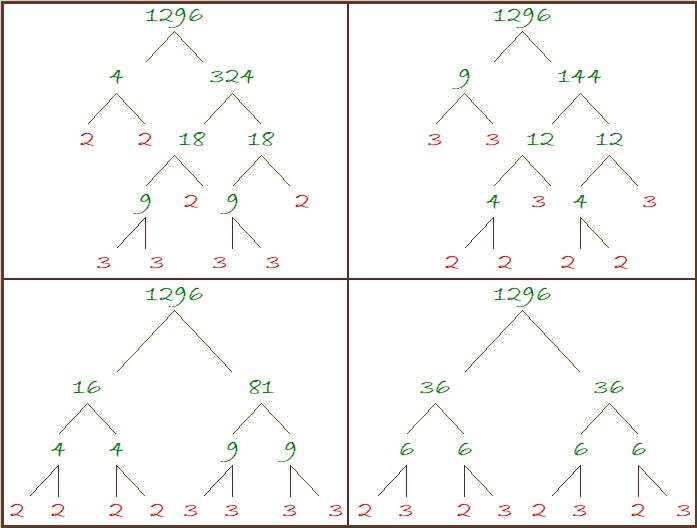1296 is the 21st Friedman number because of its digits and 9²×16 = 1296. (The same digits are on both sides of that equation.)

Since 36 is a triangular number and 36² = 1296, we get this wonderful, powerful fact:
(1 + 2 + 3 + 4 + 5 + 6 + 7 + 8)² = 1³ + 2³ + 3³ + 4³ + 5³ + 6³ + 7³ + 8³ = 1296

1296 looks interesting and often rather square when it is written in several bases:
It’s1210000 in BASE 3,
10000 in BASE 6,
2420 in BASE 8,
1700 in BASE 9,
1296 in BASE 10
900 in BASE 12,
789 in BASE 13 (Ha, ha. Seven ate nine!),
484 in BASE 17,
400 in BASE 18,
169 in BASE 33,
144 in BASE 34,
121 in BASE 35, and
100 in BASE 36

Do you know the square roots in base 10 of all the numbers in bold? Indeed, 1296 is a fascinating square number!

Here are some things I noticed on the table of powers for numbers ending in . . .

• 1: The last digit is always 1
• 2: The pattern for the last digit is 2, 4, 8, 6, repeating
• 3: The pattern for the last digit is 3, 9, 7, 1, repeating
• 4: The pattern for the last digit is 4, 6, 4, 6, repeating
• 5: The last digit is always 5
• 6: The last digit is always 6
• 7: The pattern for the last digit is 7, 9, 3, 1, repeating (3’s pattern backward but starting with 7)
• 8: The pattern for the last digit is 8, 4, 2, 6, repeating (2’s pattern backward but starting with 8)
• 9: The pattern for the last digit is 9, 1, 9, 1, repeating
• 0: The last digit is always 0

For the squares:

• 1² and 9² both end in 1, and 1 + 9 = 10
• 2² and 8² both end in 4, and 2 + 8 = 10
• 3² and 7² both end in 9, and 3 + 7 = 10
• 4² and 6² both end in 6, and 4 + 6 = 10

For the cubes:

• 1³ ends in 1, and 9³ ends in 9
• 2³ ends in 8, and 8³ ends in 2; 2 + 8 = 10
• 3³ ends in 7, and 7³ ends in 3; 3 + 7 = 10
• 4³ ends in 4, and 6³ ends in 6

Other observations:

• No matter what n is, the last digit of n, n⁵, and n⁹ are always the same. Yes, that pattern continues with n¹³ and so forth.
• The number of digits for 10ᵃ is a+1, and the number of digits for 9ᵃ is a.

Dan Bach noticed something else.

I had never heard of Benford’s Law before, so I’ve learned something new here!  All of us can learn something new by observing patterns and listening to each other.

# Reasons to Celebrate 1155

1155 is the product of the four smallest odd prime numbers. That alone is a reason to celebrate. Here, I’ve made a factor cake with 1155 for you to enjoy!make science GIFs like this at MakeaGif

Besides 1155 being the smallest number divisible by the first 4 odd prime numbers, what else can I say about it?

• 1155 is a composite number.
• Prime factorization: 1155 = 3 × 5 × 7 × 11
• The exponents in the prime factorization are 1, 1, 1, and 1. Adding one to each and multiplying we get (1 + 1)(1 + 1)(1 + 1)(1 + 1) = 2 × 2 × 2 × 2 = 16. Therefore 1155 has exactly 16 factors.
• Factors of 1155: 1, 3, 5, 7, 11, 15, 21, 33, 35, 55, 77, 105, 165, 231, 385, 1155
• Factor pairs: 1155 = 1 × 1155, 3 × 385, 5 × 231, 7 × 165, 11 × 105, 15 × 77, 21 × 55, or 33 × 35
• 1155 has no square factors that allow its square root to be simplified. √1155 ≈ 33.98529Since it’s number of prime factors is a power of 2, it can make some very well-balanced and attractive factor trees:1155 is the hypotenuse of a Pythagorean triple:
693-924-1155 which is (3-4-5) times 231

1155 is one number away from the next perfect square, AND it is the difference of two squares EIGHT different ways.
34² – 1² = 1155
38² – 17² = 1155
46² – 31² = 1155
58² – 47² = 1155
86² – 79² = 1155
118² – 113² = 1155
194² – 191² = 1155
578² – 577² = 1155

Those eight ways correspond to its eight factor pairs. Notice that both of the numbers in all of its factor pairs are odd numbers.

I like the way 1155 looks in some other bases:
It’s 3A3 in BASE 18 (A is 10 base 10) because 3(18²) + 10(18) + 3(1) = 1155
XX in BASE 34 (X is 33 base 10) because 33(34) + 33(1) = 33(35) = 1155
X0 in BASE 35 because 33(35) = 1155

XX X0 . . . Hugs and Kisses. You gotta love that!

# STOP! Look How Cool a Number 1080 Is!

### STOP Sign Geometry Fact:

What can I tell you about the number 1080?  Lots of things!

The sum of the interior angles of an eight-sided polygon such as a stop sign is 1080°.The sum of the interior angles of an octagon is 6(180°) = 1080°. Convex or Concave, it doesn’t matter, the sum of those interior angles of an eight-sided polygon will still be 1080°, as illustrated below:### Factor Trees for 1080:

Here are a couple of the MANY possible factor trees for 1080: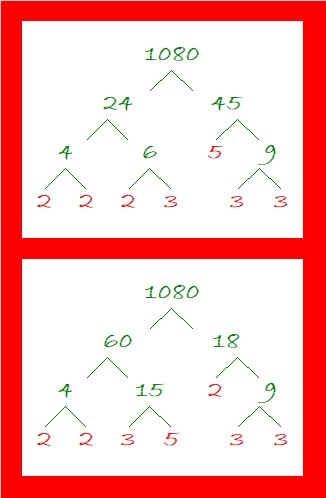### Factors of 1080:

There is only one number less than 1080 that has as many factors as 1080 does. What was that number? 840. How many factors does1080 have? 32. Wow!

• 1080 is a composite number.
• Prime factorization: 1080 = 2 × 2 × 2 × 3 × 3 × 3 × 5, which can be written 1080 = 2³ × 3³ × 5.
• The exponents in the prime factorization are 3, 3, and 1. Adding one to each and multiplying we get (3 + 1)(3 + 1)(1 + 1) ) = 4 × 4 × 2 = 32. Therefore 1080 has exactly 32 factors.
• Factors of 1080: 1, 2, 3, 4, 5, 6, 8, 9, 10, 12, 15, 18, 20, 24, 27, 30, 36, 40, 45, 54, 60, 72, 90, 108, 120, 135, 180, 216, 270, 360, 540, 1080
• Factor pairs: 1080 = 1 × 1080, 2 × 540, 3 × 360, 4 × 270, 5 × 216, 6 × 180, 8 × 135, 9 × 120, 10 × 108, 12 × 90, 15 × 72, 18 × 60, 20 × 54, 24 × 45, 27 × 40 or 30 × 36
• Taking the factor pair with the largest square number factor, we get √1080 = (√36)(√30) = 6√30 ≈ 32.863351080 has 28 composite factors and is the smallest number that can make that claim. (Of its 32 factors, all are composite numbers except 1, 2, 3, and 5). That’s more than 840’s 27 composite factors. (Its 32 factors minus 1, 2, 3, 5, and 7)

### Sum Difference Puzzles:

30 has four factor pairs. One of those pairs adds up to 13, and  another one subtracts to 13. Put the factors in the appropriate boxes in the first puzzle.

1080 has sixteen factor pairs. One of the factor pairs adds up to ­78, and a different one subtracts to 78. If you can identify those factor pairs, then you can solve the second puzzle!The second puzzle is really just the first puzzle in disguise. Why would I say that?

### Other Facts about the Number 1080:

1080 is the sum of these four consecutive prime numbers:
263 + 269 + 271 + 277 = 1080

1080 is the sum of four consecutive powers of three:
3⁶ + 3⁵ + 3⁴ + 3³ = 1080

1080 is the hypotenuse of a Pythagorean triple:
648-864-1080 which is (3-4-5) times 216

Note that 5(6³) = 5(216) = 1080 so 1080 is 500 in BASE 6.
It’s palindrome 252 in BASE 22 because 2(22²) + 5(22) + 2(1) = 1080,
UU in BASE 35 (U is 30 base 10) because 30(35) + 30(1) = 30(36) = 1080,
and it’s U0 in BASE 36 because 30(36) = 1080

And now I’ll STOP writing about how cool 1080 is.

# 1050 Factor Trees Are In Bloom This Spring

1050 has much more than the average number of factors for a number its size. I decided to make a few of its MANY possible factor trees in some springtime colors. I hope you enjoy looking at them. Its prime factors, 2, 3, 5, 5, and 7, are in green.What can I tell you about the number 1050?

• 1050 is a composite number.
• Prime factorization: 1050 = 2 × 3 × 5 × 5 × 7, which can be written 1050 = 2 × 3 × 5² × 7
• The exponents in the prime factorization are 1, 1, 2, and 1. Adding one to each and multiplying we get (1 + 1)(1 + 1)(2 + 1)(1 + 1) = 2 × 2 × 3 × 2 = 24. Therefore 1050 has exactly 24 factors.
• Factors of 1050: 1, 2, 3, 5, 6, 7, 10, 14, 15, 21, 25, 30, 35, 42, 50, 70, 75, 105, 150, 175, 210, 350, 525, 1050
• Factor pairs: 1050 = 1 × 1050, 2 × 525, 3 × 350, 5 × 210, 6 × 175, 7 × 150, 10 × 105, 14 × 75, 15 × 70, 21 × 50, 25 × 42, or 30 × 35
• Taking the factor pair with the largest square number factor, we get √1050 = (√25)(√42) = 5√42 ≈ 32.403703If you had \$10.50 in quarters, you would have 42 quarters because 42(25) = 1050
If you had \$10.50 in dimes, you would have 105 dimes because 105(10) = 1050
If you had \$10.50 in nickles, you would have 210 nickles because 210(5) = 1050

1050 is the sum of the twenty-two primes from 7 to 97.

1050 is the hypotenuse of two Pythagorean triples:
630-840-1050 which is (3-4-5) times 210
294-1008-1050 which is (7-24-25) times 42

1050 looks interesting to me when it is written in a couple of other bases:
It’s UU in BASE 34 (U is 30 base 10) because 30(34) + 30(1) = 30(35) = 1050,
and it’s U0 in BASE 35 because 30(35) = 1050

# 882 Factor Trees for the First Day of Autumn

September 22 was the first day of autumn. Leaves are already beginning to fall from the trees.

To rake up the leaves for 882, you might first notice that it’s even. The logical thing to do would be to first divide 882 by two. . . But perhaps you might notice that 8 + 8 + 2 = 18, a number divisible by nine, so you might just as logically begin by dividing 882 by 9. The first step you take determines how the factor tree looks.882 has many possible factor trees but these two are probably the most common.

You can rake the leaves up this way or you can rake them up that way, but when you rake up the leaves from 882’s factor trees, you always get the same prime factors: 2, 3, 3, 7, and 7.

Here’s a little more about the number 882:

882 has eighteen factors. The greatest number less than 882 with eighteen factors is 828. Now get this: 288 also has eighteen factors. That means that every possible combination of 8-8-2 has exactly eighteen factors!

882 has some interesting representations in some other bases:

• 616 BASE 12, because 6(12²) + 1(12)¹ + 6(12º) = 882
• 242 BASE 20, because 2(20²) + 4(20)¹ + 2(20º) = 882
• 200 BASE 21, because 2(21²) = 882

882 is also the sum of consecutive primes: 439 + 443 = 882

• 882 is a composite number.
• Prime factorization: 882 = 2 × 3 × 3 × 7 × 7, which can be written 882 = 2 × 3² × 7²
• The exponents in the prime factorization are 1, 2 and 2. Adding one to each and multiplying we get (1 + 1)(2 + 1)(2 + 1) = 2 × 3 × 3 = 18. Therefore 882 has exactly 18 factors.
• Factors of 882: 1, 2, 3, 6, 7, 9, 14, 18, 21, 42, 49, 63, 98, 126, 147, 294, 441, 882
• Factor pairs: 882 = 1 × 882, 2 × 441, 3 × 294, 6 × 147, 7 × 126, 9 × 98, 14 × 63, 18 × 49 or 21 × 42
• Taking the factor pair with the largest square number factor, we get √882 = (√441)(√2) = 21√2 ≈ 29.6984848.# 840 is the Smallest Number that Is the Top Number in THREE Sum-Difference Puzzles

### Factor Trees for 840:

840 has more factors than any previous number. Those factors will help us write 840 as the sum of consecutive counting numbers, consecutive even numbers, and consecutive odd numbers. What are the factors of 840? Here are a couple of the many possible factor trees for 840:The red leaves on the tree are prime numbers. Gathering the six red leaves from either factor tree above gives us 840’s prime factorization: 840 = 2³ × 3 × 5 × 7.  Now 840 is not the smallest number to have six red leaves. In fact, there are smaller numbers with as many as nine leaves, but 840’s six innocent-looking red leaves will turn into a whopping 32 factors!

### Factors of 840:

• 840 is a composite number.
• Prime factorization: 840 = 2 × 2 × 2 × 3 × 5 × 7, which can be written 840 = 2³ × 3 × 5 × 7.
• The exponents in the prime factorization are 3, 1, 1, and 1. Adding one to each and multiplying we get (3 + 1)(1 + 1)(1 + 1) )(1 + 1) = 4 × 2 × 2 × 2 = 32. Therefore 840 has exactly 32 factors.
• Factors of 840: 1, 2, 3, 4, 5, 6, 7, 8, 10, 12, 14, 15, 20, 21, 24, 28, 30, 35, 40, 42, 56, 60, 70, 84, 105, 120, 140, 168, 210, 280, 420, 840
• Factor pairs: 840 = 1 × 840, 2 × 420, 3 × 280, 4 × 210, 5 × 168, 6 × 140, 7 × 120, 8 × 105, 10 × 84, 12 × 70, 14 × 60, 15 × 56, 20 × 42, 21 × 40, 24 × 35 or 28 × 30
• Taking the factor pair with the largest square number factor, we get √840 = (√4)(√210) = 2√210 ≈ 28.98275.840 is also the smallest number that can be evenly divided by the first eight counting numbers!

### Sum-Difference Puzzles:

210 has eight factor pairs. One of those factor pairs adds up to 29, and another one subtracts to 29. Another factor pair adds up to 37, and a different one subtracts to 37. Thus, 210 produces two different Sum-Difference Puzzles shown in the first two graphics below!

840 is a multiple of 210 and  has sixteen factor pairs! One of the factor pairs adds up to ­58 and another to 74. A different factor pair subtracts to 58, and still a different one subtracts to 74. If you can identify those factor pairs, then you can solve the two puzzles that are next to the 210 puzzles below!

###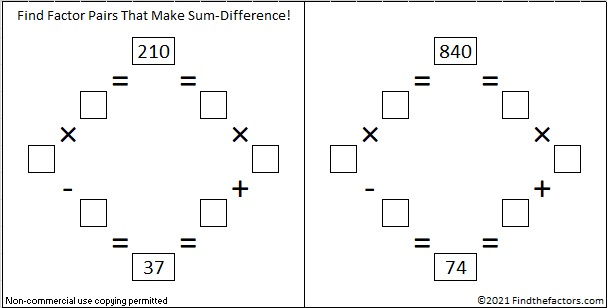840 has yet another factor pair that adds up to 113 and a different one that subtracts to 113. If you can find those factor pairs, then you can solve this next primitive puzzle:If you need help with any of those 840 Sum-Difference puzzles, the chart below shows the sums and differences of all of 840’s factor pairs. See which sums also appear in the difference column.### How to find consecutive counting numbers that add up to 840:

840 is more than the 40th triangular number (820) and less than the 41st triangular number (861). We can also arrive at the number 40 by using √(1 + 840×2) – 1 = 40, no rounding necessary. 840 has six odd factors that are not more than 40, namely 1, 3, 5, 7, 15, 21 and 35.

I’ll describe the ways we can write 840 as the sum of consecutive numbers. Can you write out the sums? I’ve done one of them for you:

• using 3 numbers with 280 as the middle number,
• using 5 numbers with 168 as the middle number,
• using 7 numbers with 120 as the middle number; 117 + 118 + 119 + 120 + 121 + 122 + 123 = 840
• using 15 numbers with 56 as the middle number,
• using 21 numbers with 40 as the middle number
• using 35 numbers with 24 as the middle number.

Notice each of those ways has a factor pair of 840 in the description.

The largest power of 2 that is a factor of 840 is 8, which doubled becomes 16. Which of 840’s odd factors multiplied by 16 are not more than 40? 1 × 16 = 16, and 3 × 16 = 48. Oops, that’s too much. The rest of its odd factors times 16 will be too much as well. Nevertheless, we can write 840 as the sum of 16 counting numbers. 840÷16 = 52.5 so 52 and 53 will be the 8th and 9th numbers in the sum.

• 45 + 46 + 47 + 48 + 49 + 50 + 51 + 52 + 53 + 54 + 55 + 56 + 57 + 58 + 59 + 60 = 840

### How do we find consecutive EVEN numbers that add up to an even number?

Only even numbers can be the sum of consecutive even numbers. Let’s use 840 as an example again. 840÷2 = 420.First, we will find all the ways to write 420 as the sum of consecutive numbers. Then we will simply double the middle number and surround it with the appropriate number of even numbers to get a sum of even numbers that add up to 840:

√(1 + 420×2) – 1 = 28, no rounding necessary, so we will make a list of the odd factors that are not more than 28. They are 1, 3, 5, 7, 15, and 21. We also note that the largest power of 2 that is a factor of 420 is 4. Doubling 4, we get 8. Which of 420’s odd factors multiplied by 8 are not more than 28? 1 × 8 = 8, and 3 × 8 = 24. All of the rest will be too much.

I’ll describe the ways we can write 840 as the sum of consecutive even numbers. You can see 420’s factor pairs and 840’s factor pairs in the descriptions. Can you write out the sums? I’ve done one of them for you:

• using 3 even numbers with 2 × 140 = 280 as the middle number
• using 5 even numbers with 2 × 84 = 168 as the middle number
• using 7 even numbers with 2 × 60 = 120 as the middle number
• using 15 even numbers with 2 × 28 = 56 as the middle number
• using 21 even numbers with 2 × 20 = 40 as the middle number; 20 + 22 + 24 + 26 + 28 + 30 + 32 + 34 + 36 + 38 + 40 + 42 + 44 + 46 + 48 + 50 + 52 + 54 + 56 + 58 + 60 = 840

We can also write 840 as the sum of an even amount of consecutive even numbers.

• using 8 even numbers: 2 times (49 + 50 + 51 + 52 + 53 + 54 + 55 + 56 = 420) becomes 98 + 100 + 102 + 104 + 106 + 108 + 110 + 112 = 840. Notice that 840÷8 = 105, the odd number that is between the two numbers in the exact middle of the sum.
• Likewise, using 24 even numbers: 12 + 14 + 16 + 18 + 20 + 22 + 24 + 26 + 28 + 30 + 32 + 34 + 36 + 38 + 40 + 42 + 44 + 46 + 48 + 50 + 52 + 54 + 56 + 58 = 840. Notice that 840÷ 24 = 35, the odd number that is between the two numbers in the exact middle of the sum.

### How to find consecutive ODD numbers that add up to an even number like 841:

Let me first point out that every square number, n², is the sum of the first n numbers.

For example: 100 = 10², and 100 is also the sum of the first 10 odd numbers as illustrated below: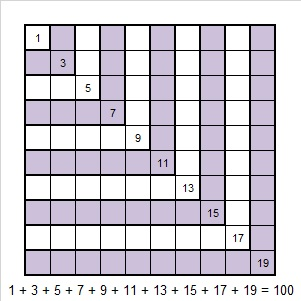A similar statement is true for every other square number:Yes, every square number, n², is the sum of the first n odd numbers.

To write a non-square even number as the sum of consecutive odd numbers, the first thing we must do is determine if the number can be written as the difference of two squares. If an even number has a factor pair, b × a, in which b > a, and BOTH a and b are even, then that even number can be expressed as the difference of two squares by using [(b + a)/2]² – [(b – a)/2]².

Now as long as an even number can be expressed as the difference of two squares, , then that number can be written as the sum of all the odd numbers from 2A+1 to 2B-1.

840 is an even number with eight factor pairs in which both numbers are even. Let’s use those even factor pairs to find the ways to write 840 as the sum of consecutive ODD numbers:

• 420 × 2 = 840 means 211² 209² = 840, and that means the sum of the 2 consecutive odd numbers from 419 to 421 = 840
• 210 × 4 = 840 means 107² 103² = 840, and that means the sum of the 4 consecutive odd numbers from 207 to 213 = 840
• 140 × 6 = 840 means 73² 67² = 840, and that means the sum of the 6 consecutive odd numbers from 135 to 145 = 840
• 84 × 10 = 840 means 47² 37² = 840, and that means the sum of the 10 consecutive odd numbers from 75 to 93 = 840
• 70 × 12 = 840 means 41² 29² = 840, and that means the sum of the 12 consecutive odd numbers from 59 to 81 = 840
• 60 × 14 = 840 means 37² 23² = 840, and that means the sum of the 14 consecutive odd numbers from 47 to 73 = 840
• 42 × 20 = 840 means 31² 11² = 840, and that means the sum of the 20 consecutive odd numbers from 23 to 61 = 840
• 30 × 28 = 840 means 29² = 840, and that means the sum of the 28 consecutive odd numbers from 3 to 29 = 840

Thus, 840 with its record setting 32 factors, can be written as the sum of 7 consecutive numbers, 7 consecutive even numbers, and 8 consecutive odd numbers!

### More about the Number 840:

Incidentally, being able to write 840 as the difference of two squares, eight different ways also makes 840 a leg in at least eight different Pythagorean triples. Those Pythagorean triples can be calculated using the numbers from the difference of two squares. For example, 682-840-1082 can be calculated from 2(31)(11), 31² 11², 31² + 11².

840 was the leg for those eight triples. It is possible that looking at 2(b)(a), where b × a = 420, will produce some more Pythagorean triples with 840 as the leg.

840 is also the hypotenuse of one Pythagorean triple, 504-672-840.

On a note totally unrelated to anything I’ve written above, 840 is a repdigit in two bases:

• SS BASE 29 (S is 28 base 10) Note that 28(29) + 28(1) = 28(30) = 840
• 00 BASE 34 (0 is 24 base 10) Note that 24(34) + 24(1) = 24(35) = 840

840 is also the sum of twin prime numbers 419 and 421.

# 780 is the 39th Triangular Number

• 780 is a composite number.
• Prime factorization: 780 = 2 x 2 x 3 x 5 x 13, which can be written 780 = (2^2) x 3 x 5 x 13
• The exponents in the prime factorization are 2, 1, 1, and 1. Adding one to each and multiplying we get (2 + 1)(1 + 1)(1 + 1)(1 + 1) = 2 x 3 x 2 x 2 = 24. Therefore 780 has exactly 24 factors.
• Factors of 780: 1, 2, 3, 4, 5, 6, 10, 12, 13, 15, 20, 26, 30, 39, 52, 60, 65, 78, 130, 156, 195, 260, 390, 780
• Factor pairs: 780 = 1 x 780, 2 x 390, 3 x 260, 4 x 195, 5 x 156, 6 x 130, 10 x 78, 12 x 65, 13 x 60, 15 x 52, 20 x 39, or 26 x 30
• Taking the factor pair with the largest square number factor, we get √780 = (√4)(√195) = 2√195 ≈ 27.92848.There are MANY ways to make factor trees for 780. Here are just three of them:OEIS.org informs us that (7 + 5)(8 + 5)(0 + 5) = 780.

780 is the sum of consecutive prime numbers two different ways:

• 59 + 61 + 67 + 71 + 73 + 79 + 83 + 89 + 97 + 101 = 780 (10 consecutive primes).
• 191 + 193 + 197 + 199 = 780 (4 of my favorite consecutive primes).

26 and 30 are both exactly 2 numbers away from their average 28, so 780 can be written as the difference of two squares:

780 = 26 x 30 = (28 – 2)(28 + 2) = 28² – 2² = 784 – 4.

(It can be written as the difference of two squares three other ways, but I won’t list them here.)

Because 5 and 13 are both factors of 780, it is the hypotenuse of FOUR Pythagorean triangles making each of these equations true:

• 192² + 756² = 780²
• 300² + 720² = 780²
• 396² + 672² = 780²
• 468² + 624² = 780²

780 can be written as the sum of three perfect squares two different ways:

• 26² + 10² + 2² = 780
• 22² + 14² + 10² = 780

780 BASE 10 is palindrome QQ BASE 29. (Q is 26 in BASE 10.) Note that 26(29) + 26(1) = 780.

1 + 2 + 3 + 4 + 5 + 6 + 7 + 8 + 9 + 10 + 11 + 12 + 13 + 14 + 15 + 16 + 17 + 18 + 19 + 20 + 21 + 22 + 23 + 24 + 25 + 26 + 27 + 28 + 29 + 30 + 31 + 32 + 33 + 34 + 35 + 36 + 37 + 38 + 39 = 780, making 780 the 39th triangular number. Since 20 is in the exact middle of that list of numbers, 780 is also the 20th hexagonal number.

Triangular numbers are interesting, but are they good for anything? Here’s one good thing: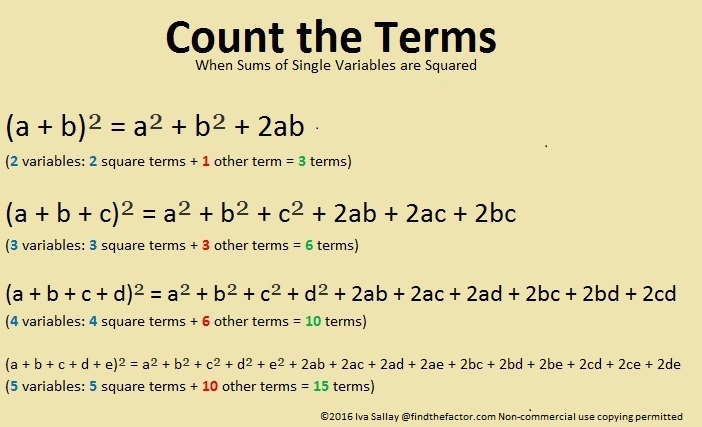There are 26 letters in the English alphabet. 39 variables would use the alphabet exactly one and one half times, but that’s okay because we can use upper case letters the second time around:

(a + b + c + . . . + x + y+ z + A + B + C + . . . + K + L + M)² has exactly 780 terms because it has 39 single variables, and 780 is the 39th triangular number.

780 is the 39th triangular number because 39⋅40/2 = 780.

A couple of months ago I saw a fascinating image on twitter. It is a square made with 1001 dots representing the numbers from -500 to +500 with zero in the exact middle. The triangular numbers are represented by the “+” pattern seen here. If we added about 600 more dots to the square, -780 and +780 would also lie on that +.